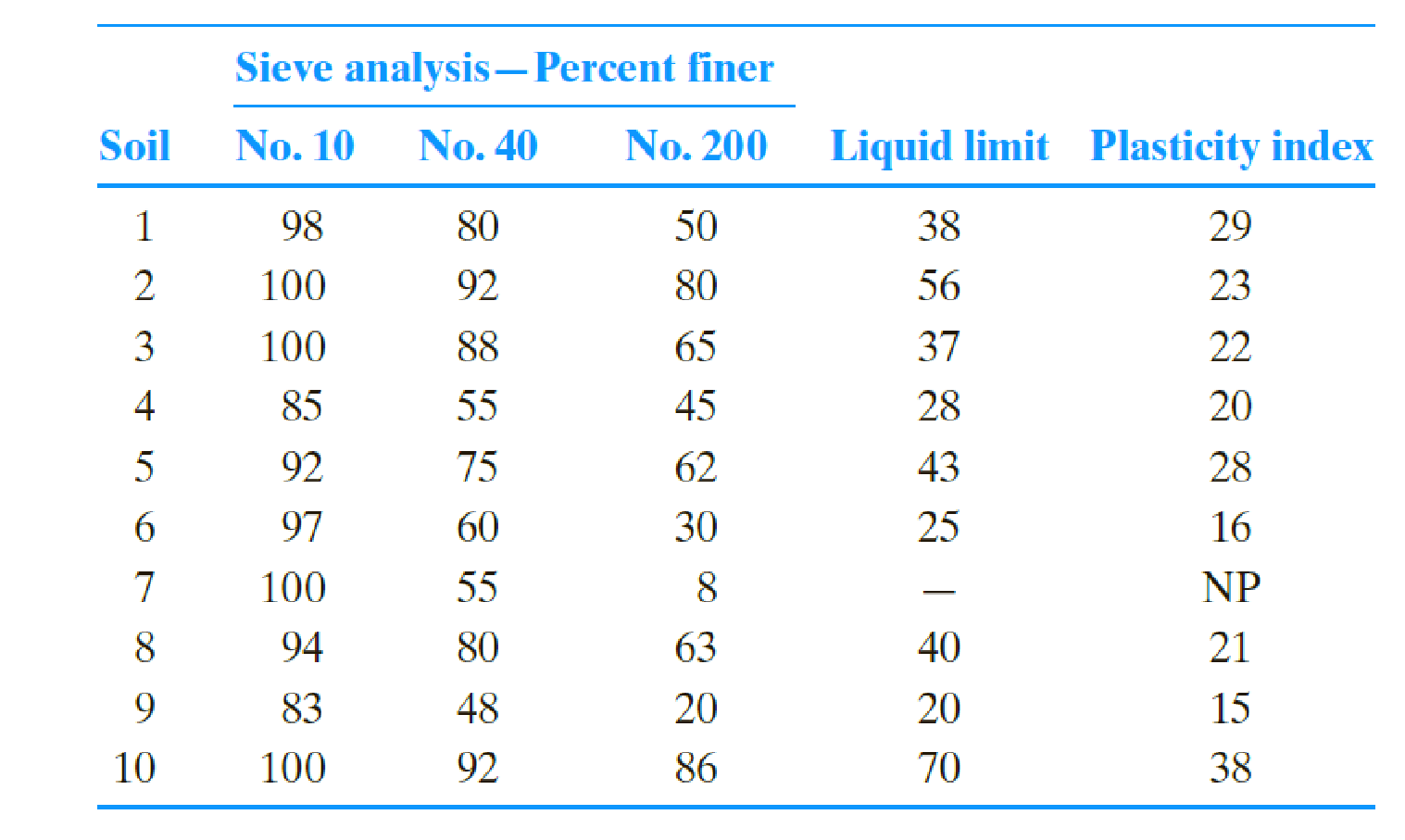Chapter 5, Problem 5.4PPrinciples of Geotechnical Enginee...

9th Edition
Braja M. Das + 1 other
ISBN: 9781305970939

Solutions

Chapter
SectionPrinciples of Geotechnical Enginee...

9th Edition
Braja M. Das + 1 other
ISBN: 9781305970939
Textbook Problem

The sieve analysis of ten soils and the liquid and plastic limits of the fraction passing through the No. 40 sieve are given below. Classify the soils by the AASHTO classification system and give the group index for each soil.To determine

The classification of each soil based on AASTHO classification and provides the group index for each soil.

Explanation

For soil 1:

Determine the group index of soil 1 using the formula.

GI=(F20035)[0.2+0.005(LL40)]+0.01(F20015)(PI10)

Here, F200 is the percentage passing through sieve No. 200, LL is the liquid limit, and PI is the plasticity index.

Refer given data.

Substitute 50 for F200, 38 for LL, and 29 for PI.

GI=(5035)[0.2+0.005(3840)]+0.01(5015)(2910)=2.85+6.65=9.510

Thus, the group index of soil 1 is 10_.

Refer Table (9.1), “Classification of highways subgrade materials” in the text book.

Show the parameter specifications from Table (5.1), and parameters of the given soil as in Table 1.

 Parameter Specification in Table (5.1) Parameters of the given soil Percent passing sieve No. 10 - 98 No. 40 - 80 No. 200 36 min 50 Liquid limit 40 max 38 Plasticity index (PI) 10 max 10

Refer Table 1.

The soil falls under the classification of A-4.

Hence, the classification of the soil as per AASTHO is A-4(10)_ since the group index of the soil is 10.

For soil 2:

Determine the group index of soil 2 using the formula.

GI=(F20035)[0.2+0.005(LL40)]+0.01(F20015)(PI10)

Refer given data.

Substitute 80 for F200, 56 for LL, and 23 for PI.

GI=(8035)[0.2+0.005(5640)]+0.01(8015)(2310)=12.6+8.45=21

Thus, the group index of soil 2 is 21_.

Show the parameter specifications from Table (5.1), and parameters of the given soil as in Table 2.

 Parameter Specification in Table (5.1) Parameters of the given soil Percent passing sieve No. 10 - 100 No. 40 - 92 No. 200 36 min. 80 Liquid limit 41 min 56 Plasticity index (PI) 11 min 23

Refer Table 2.

The soil falls under the classification of A-2-7.

Hence, the classification of the soil as per AASTHO is A-2-7(21)_ since the group index of the soil is 21.

For soil 3:

Determine the group index of soil 3 using the formula.

GI=(F20035)[0.2+0.005(LL40)]+0.01(F20015)(PI10)

Refer given data.

Substitute 65 for F200, 37 for LL, and 22 for PI.

GI=(6535)[0.2+0.005(3740)]+0.01(6515)(2210)=5.5+6=11.512

Thus, the group index of soil 3 is 12_.

Show the parameter specifications from Table (5.1), and parameters of the given soil as in Table 3.

 Parameter Specification in Table (5.1) Parameters of the given soil Percent passing sieve No. 10 - 100 No. 40 - 88 No. 200 36 min 65 Liquid limit 40 max 37 Plasticity index (PI) 11 min 22

Refer Table 3.

The soil falls under the classification of A-4.

Hence, the classification of the soil as per AASTHO is A-4(12)_ since the group index of the soil is 12.

For soil 4:

Determine the group index of soil 4 using the formula.

GI=(F20035)[0.2+0.005(LL40)]+0.01(F20015)(PI10)

Refer given data.

Substitute 45 for F200, 28 for LL, and 20 for PI.

GI=(4535)[0.2+0.005(2840)]+0.01(4515)(2010)=1.4+3=4.44.0

Thus, the group index of soil 4 is 4_.

Show the parameter specifications from Table (5.1), and parameters of the given soil as in Table 4.

 Parameter Specification in Table (5.1) Parameters of the given soil Percent passing sieve No. 10 - 85 No. 40 - 55 No. 200 36 min 45 Liquid limit 40 max 28 Plasticity index (PI) 10 max 4

Refer Table 4.

The soil falls under the classification of A-4.

Hence, the classification of the soil as per AASTHO is A-4(4)_ since the group index of the soil is 4.

For soil 5:

Determine the group index of soil 5 using the formula.

GI=(F20035)[0.2+0.005(LL40)]+0.01(F20015)(PI10)

Refer given data.

Substitute 62 for F200, 43 for LL, and 28 for PI.

GI=(6235)[0.2+0.005(4340)]+0.01(6215)(2810)=5.805+8.46=14.2714

Thus, the group index of soil 5 is 14_

Still sussing out bartleby?

Check out a sample textbook solution.

See a sample solution

The Solution to Your Study Problems

Bartleby provides explanations to thousands of textbook problems written by our experts, many with advanced degrees!

Get Started